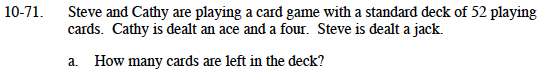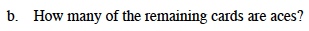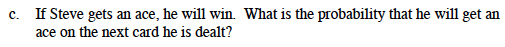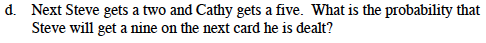### Home > MC1 > Chapter 10 > Lesson 10.2.1 > Problem10-71

10-71.There were 52 cards to begin with and 3 have been dealt. How many are left?In a standard deck, there are 4 aces. One of them has been dealt.Make the number of aces left the numerator and the number of cards left the denominator.

$\frac{3}{49}$How many cards are left in the deck? How many of them are nines?

$\frac{4}{47}$